# History of Rutherford Experiment

In Ernest Rutherford's laboratory, Hans Geiger and Ernest Marsden (a 20 yr old undergraduate student) carried out experiments to study the scattering of alpha particles by thin metal foils. In 1909 they observed that alpha particles from radioactive decays occasionally scatter at angles greater than 90°, which is physically impossible unless they are scattering off something more massive than themselves. This led Rutherford to deduce that the positive charge in an atom is concentrated into a small compact nucleus. During the period 1911-1913 in a table-top apparatus, they bombarded the foils with high energy alpha particles and observed the number of scattered alpha particles as a function of angle. Based on the Thomson model of the atom, all of the alpha particles should have been found within a small fraction of a degree from the beam, but Geiger and Marsden found a few scattered alphas at angles over 140 degrees from the beam. Rutherford's remark "It was quite the most incredible event that ever happened to me in my life. It was almost as incredible as if you had fired a 15-inch shell at a piece of tissue paper and it came back and hit you." The scattering data was consistent with a small positive nucleus which repelled the incoming positively charged alpha particles. Rutherford worked out a detailed formula for the scattering (Rutherford formula), which matched the Geiger-Marsden data to high precision.

The source used in the Rutherford experiments was purifed radium contained in a thin-walled 1-mm diameter glass tube. The source strength was about 0.1 Curie, or about 4 billion nuclear decays per second. The alpha particles were allowed to pass through a small diaphragm and were directed toward a thin foil target. The detector was a small (10-6 m2) zinc sulfide screen mounted a few centimeters away from the target. (Rohlf)

Index

Rutherford concepts

References
Rohlf
Ch 6

Blatt
Ch 5

 HyperPhysics***** Nuclear R Nave
Go Back

# Geiger-Marsden Data on Alpha ScatteringGeiger and Marsden showed that the number of scattered alpha particles as a function of scattering angle was consistent with a small, concentrated positive nucleus. To angles above 140 degrees, the nucleus appeared as a point positive charge, so this data did not measure the nuclear size. When the scattering departed from that predicted from Coulomb's law, it could be inferred that another force was coming into play and you could claim to have "hit" the nucleus. At 140 degrees, they still hadn't hit it.

 Sketch of experiment

Since they had used the most energetic alpha particles at their disposal, 7.7 MeV, and still hadn't seen the departure from Coulomb scattering which would signal a direct interaction with the nucleus, they had to look for other alternatives to get a measure of nuclear size. This led to a famous remark by Rutherford to his graduate students "There is no money for apparatus -. we shall have to use our heads!"(Keller) The form that "using their heads" took was the study of lighter target nuclei, and finally the use of aluminum target nuclei yielded measurable direct backscatter of alpha particles, at least establishing an upper bound for the nuclear size.

 What can you learn from backscatter from aluminum?
Index

Rutherford concepts

Scattering concepts

 HyperPhysics***** Nuclear R Nave
Go Back

# Conditions for large angle scattering

Regardless of the nature of the force in an elastic collision, to get a scattering angle greater than 90 degrees, the target must be more massive than the projectile.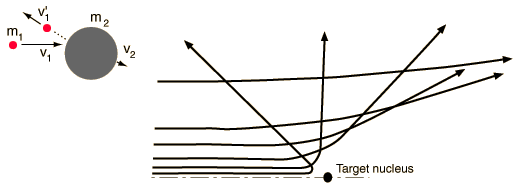Index

Rutherford concepts

Scattering concepts

Beiser reference

 HyperPhysics***** Nuclear R Nave
Go Back

# Determining the Impact Parameter

Besides the masses of the target and projectile, the scattering angle depends upon the force and upon the impact parameter. The impact parameter is the perpendicular distance to the closest approach if the projectile were undeflected.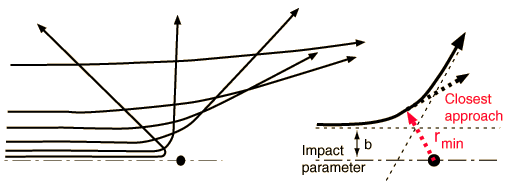For coulomb scattering of a small projectile off a massive nucleus, the impact parameter is related to the scattering angle by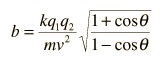Show For the special case of alpha scattering from a nucleus of atomic number Z, the relationship is more convenient in the form to the right.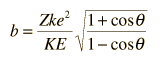The constant multiplying Z has the value 1.44 MeV fm in units which are compatible with kinetic energies expressed in MeV. It should be noted that the kinetic energy expression used is non-relativistic, so that the expression must be modified for the extremely high energies obtained in accelerators.
For alpha particle scattering off a nucleus of atomic number Z =, an observed scattering angle of degrees for an alpha particle of kinetic energy MeV corresponds to an impact parameter of
b = x10^ m = fermi.

Determining the closest approach to the nucleus amounts to calculating the minimum distance for the hyperbolic orbit which is produced by the coulomb repulsive force. The expression for the closest approach as a function of the impact parameter b is given by: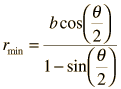Which has a limit for back-scattering which is just determined by setting the initial kinetic energy equal to the final potential energy. This corresponds to stopping the projectile and sending it backward, so at its closest approach, all the energy is in electric potential energy.The closest approach for the trajectory described above is then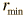=x10^ m = fermi.

Rutherford scattering was the first method used to measure the size of nuclei. More precise measurements are made with electron scattering, and it was discovered that the density of nuclei is approximately constant. This has made possible the modeling of nuclear radii from just their mass numbers. As a comparison for the calculated parameters above, if we assume a mass number of twice Z above, an approximate nuclear radius can be calculated.

estimated nuclear radius for A=2Z is r = fermi.

This will always be an underestimate because of the neutron excess of heavy nuclei, but a more accurate value can be obtained by using the nuclear mass in the nuclear radius relationship.

The default calculation above will be the calculation of the impact parameter b and the radius of closest approach. However, if you substitute a number for the radius of closest approach, it will calculate the kinetic energy necessary to produce that value, given the angle and other parameters.

 Relationship of impact parameter to scattering cross section
Index

Rutherford concepts

Scattering concepts

Beiser reference

Fowles reference

 HyperPhysics***** Nuclear R Nave
Go Back